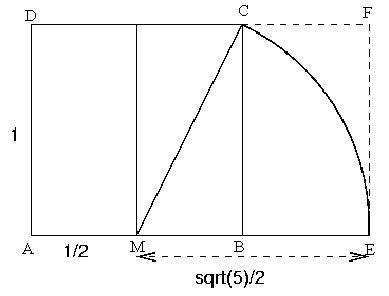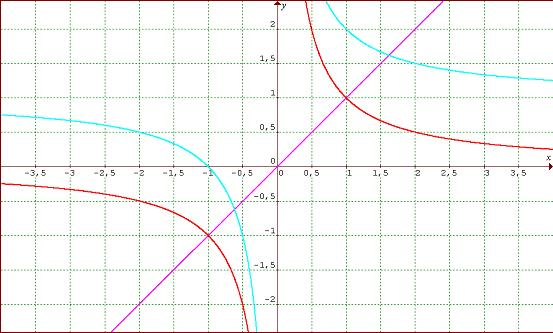#### You may also like### Gold Again

Without using a calculator, computer or tables find the exact values of cos36cos72 and also cos36 - cos72.### Pythagorean Golden Means

Show that the arithmetic mean, geometric mean and harmonic mean of a and b can be the lengths of the sides of a right-angles triangle if and only if a = bx^3, where x is the Golden Ratio.### Golden Triangle

Three triangles ABC, CBD and ABD (where D is a point on AC) are all isosceles. Find all the angles. Prove that the ratio of AB to BC is equal to the golden ratio.

# Golden Construction

##### Age 16 to 18 Challenge Level:Thank you to Shaun from Nottingham High School and Andrei from Tudor Vianu National College, Bucharest, Romania for these solutions.

(1) Drawing the figure, I observe that ratios $AE/AD$ and $BC/BE$ are approximately equal, having a value of 1.6.

(2) From Pythagoras' Theorem I calculate $MC$ (in the right-angled triangle $MBC$): \eqalign{ MC^2 &= BC^2 + MB^2 = 1 + 1/4 \cr MC &= \sqrt5 /2 }. So $AE=(\sqrt 5 + 1)/2$ and $BE=(\sqrt 5 - 1)/2$. The ratios are: $${AE\over AD}= {\sqrt 5 + 1\over 2}$$ and $${BC\over BE}= {1\over (\sqrt 5 - 1)/2} = {\sqrt 5 + 1\over 2}.$$ So, $AE/AD = BC/BE.$

(3) From this equality of ratios, I find out that $$BE = {AD.BC\over AE} = {1\over \phi}$$ But $AE = AB + BE$ so $$\phi = 1 + {1\over \phi}.$$(4) Substituting $\phi = 1$ the left hand side of this expression is less than the right hand side. If we increase the value given to $\phi$ the left hand side increases and the right hand side decreases continuously. Substituting $\phi = 2$ the left hand side is greater than the right hand side so the value of $\phi$ which satisfies this equation must lie between $1$ and $2$.

The two solutions of the equation can be found at the intersection of the cyan curve ($y=1 + 1/x$) and magenta curve ($y=x)$. Only the positive value is considered and it is approximately 1.618.

(5) Now, I solve the equation. It is equivalent to $\phi^2 - \phi -1 = 0$ so the solutions are $$\phi_1 = {1-\sqrt 5\over 2}$$ and $$\phi_1 = {1+\sqrt 5 \over 2}.$$ Only the second solution is valid because $\phi > 0$ .